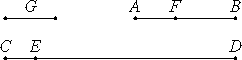# Proposition 3

To find the greatest common measure of two given commensurable magnitudes.

Let the two given commensurable magnitudes be AB and CD with AB the less.

It is required to find the greatest common measure of AB and CD.

Now the magnitude AB either measures CD or it does not.

If it measures it, and it does measures itself, then AB is a common measure of AB and CD. And it is manifest that it is also the greatest, for a greater magnitude than the magnitude AB does not measure AB.Next, let AB not measure CD.

X.2

Then, if the less is continually subtracted in turn from the greater, then that which is left over will sometime measure the one before it, because AB and CD are not incommensurable.

Let AB, measuring ED, leave EC less than itself, let EC, measuring FB, leave AF less than itself, and let AF measure CE.

Since, then, AF measures CE, while CE measures FB, therefore AF also measures FB. But it measures itself also, therefore AF also measures the whole AB. But AB measures DE, therefore AF also measures ED. But it measures CE also, therefore it also measures the whole CD.

Therefore AF is a common measure of AB and CD.

I say next that it is also the greatest.

If not, then there there is some magnitude G greater than AF which measures AB and CD. Since then G measures AB, while AB measures ED, therefore G also measures ED.

But it measures the whole CD also, therefore G measures the remainder CE. But CE measures FB, therefore G also measures FB.

But it measures the whole AB also, and it therefore measures the remainder AF, the greater the less, which is impossible.

Therefore no magnitude greater than AF measures AB and CD. Therefore AF is the greatest common measure of AB and CD.

Therefore the greatest common measure of the two given commensurable magnitudes AB and CD has been found.

Q.E.D.

# Corollary.

From this it is clear that, if a magnitude measures two magnitudes, then it also measures their greatest common measure.

## Guide

This is the same proposition as VII.3 with the same diagram and the same corollary, only the terminology is slightly different.

This proposition and its corollary are used in the next proposition.